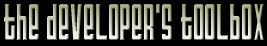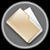This section of the archives stores flipcode's complete Developer Toolbox collection, featuring a variety of mini-articles and source code contributions from our readers.Reducing Indentation In Code   Submitted byThis tip will work for any language, although I'll use C++ in the examples.

While reading/fixing some Winsock 2 code written by Intel so that I could use it myself, I realized that they used absurd amounts of nested if statements for error checking. For example...

 ```void install() { bool result = first(); if (result) { result = second(); if (result) { result = third(); if (result) { result = fourth(); if (result) { installed = true; } // if } // if } // if } // if } // void install() ```

Deeply nested code is typically difficult to read since your brain has to keep track of many control paths.

For example, here is a control path graph based on the code above:
```        o
/ \
/   o
/   / \
/   /   o
/   /   / \
/   /   /   o
/   /   /   / \
o   o   o   o   o
```

Now let's make that code above much more readable.

 ```void install() { bool result = first(); if (!result) { return; } result = second(); if (!result) { return; } result = third(); if (!result) { return; } result = fourth(); if (!result) { return; } installed = true; } // void install() ```

This code has a more linear feel (although some people consider return and break similar to goto, and therefore a Bad Thing) and is now broken into logical steps. The returns can be replaced with some exception throws, if you want.

The reason the code is easier to read is because some control paths terminate early, rather than lasting until the end of the function is hit.

Here is the new control path graph:

```  o
/ \
o   o
/ \
o   o
/ \
o   o
/ \
o   o
```

You see? Most of you probably think this is obvious, but since whoever at Intel wrote the Winsock 2 Layered Service Provider example code didn't, I thought it might be good to tell everyone. ^_^

--